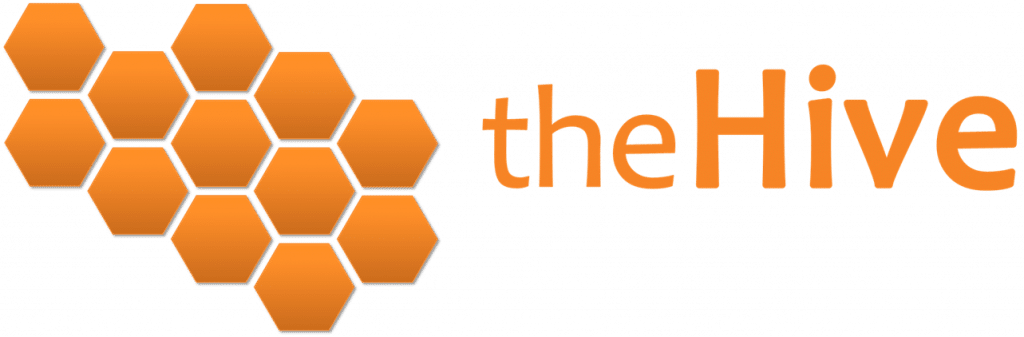## ​Binomial Distribution - Do You Know How to Get One?

The Binomial Distribution can be a bit of a mystery.

How can you get one just from flipping a coin? Where does it come from? And ​what can you do with it?

Well, the mathematics behind the binomial distribution may be difficult to understand, but it's actually straight-forward in how it comes about.

Don't believe me?

Then stick around and I'll prove it to you.

For a while now I've been researching all the little nooks and crannies of the statistics world to create a big picture of what it looks like when you pull it all together.

And this is what the part about ​Probability Distributions looks like: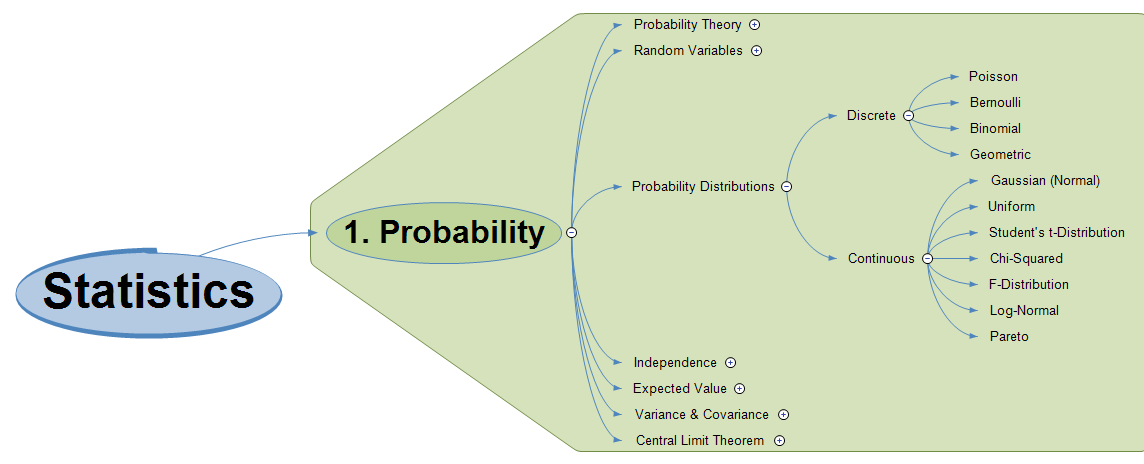​And to help you understand more about Probability Distributions in general, I'm going to show you exactly how the Binomial Distribution comes about.

### Choices, Choices...

And now I'm going to give you a choice...

Ooh, I like choices!

It's up to you...

### Introduction to ​the Binomial Distribution

​Most people understand that if you toss a coin, the probability of getting a Head is 1/2, and the probability of getting a Tail is also 1/2.

There are two possible outcomes and the chances of each are identical.

So how does that make any kind of distribution, let alone a binomial distribution?

To understand that, I'm going to build a story ​in 5 steps so you can see how all the magic happens.

We're going to look at:

1. 1
​Tossing a single coin
2. 2
​Tossing ​2 coins simultaneously
3. 3
​Tossing ​10 coins simultaneously
4. 4
​Plotting a Histogram from the results
5. 5
​Creating a binomial distribution from the Histogram

And just so you know, this video lesson is actually one of the lessons in The Hive from our exclusive video course Statistics - The Big Picture, which is Open Access to start with (you don't need to register).

If you want to continue learning, you can start from the beginning here:

### Statistics - The Big Picture

#### 1. ​Tossing a Single Coin

​So we know that tossing a single coin will give us either a Head or a Tail, and each has the probability of 1/2. Laying out all possible outcomes will give us something like this: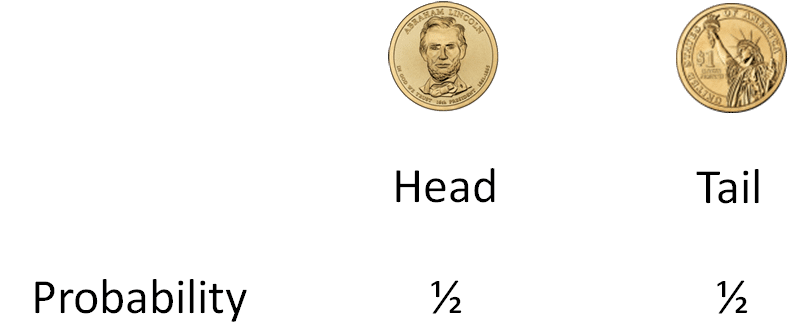​And here is where the confusion usually starts, because there is no kind of distribution here - all the possible outcomes are identical.

To start to understand where our binomial distribution might come from, we need to look at what happens when we have 2 coins.#### Pin it for later

Need to save this for later?

Pin it to your favourite board  and you can get back to it when you're ready.

#### 2. ​Tossing 2 Coins

​So now let's toss a pair of coins and see what happens.

This time, there are 4 possible outcomes. We might get a pair of Heads (HH), a Head and a Tail (HT), a Tail and a Head (TH) or a pair of Tails (TT), like this: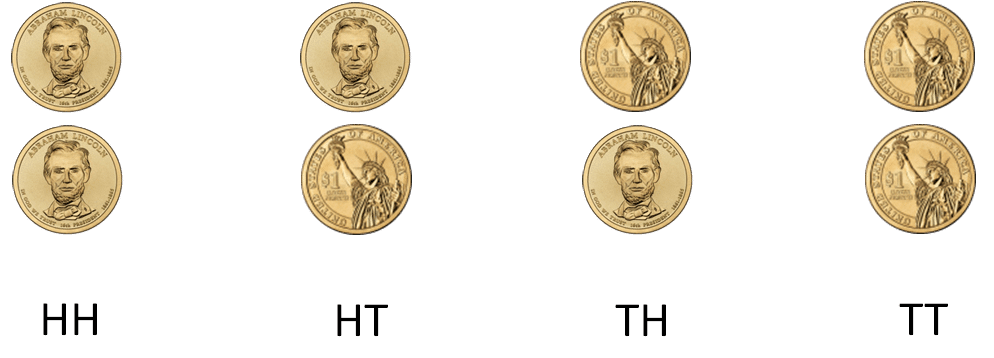​We know what the individual probabilities are for Heads and Tails, and if we get a Head AND another Head, we can multiply their individual probabilities together to get the probability that both outcomes (a pair of Heads) happen together. So 1/2 x 1/2.

We can do this for all possible outcomes and we get this:​Still not seeing any kind of distribution yet, but the next part is where the magic happens!

Take a look at the middle two results. HT and TH.

Do we care which order the results came out?

Nope.

So they're the same result aren't they?

Yup.

​So we can ​group them together!

This is the important bit, so pay attention! In the middle we have two outcomes that are the same, so we can ​group them together, and by doing this we can ADD together their probabilities, like this: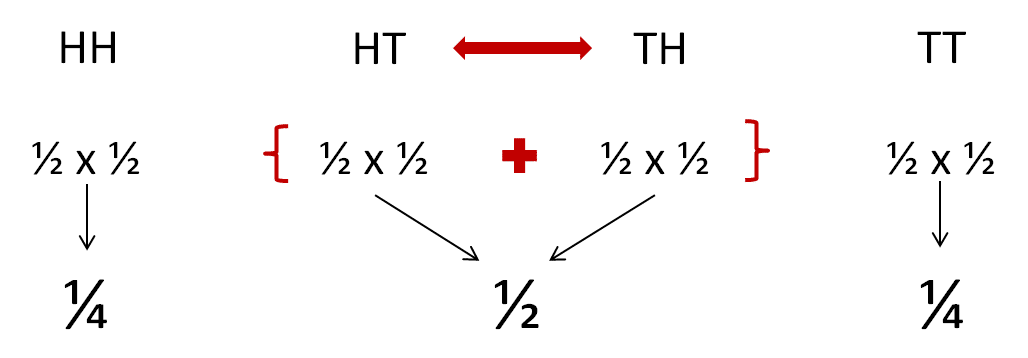​So now we have a probability in the middle that is larger than the probabilities at either end.

It's not a distribution, but it has the makings of one!

Binomial Distribution - ​Do You Know How to Get One? (video) #probability #binomial @chi2innovations @eelrekab

#### 3. ​Tossing 10 Coins

​Now let's toss 10 coins repeatedly to see what happens.

For this, we're going to start by creating an x-axis for all possible outcomes.

At the left we have a bin that corresponds to tossing 10 Heads and 0 Tails. Every time we get that outcome we add a token to the left-most bin.

Similarly, whenever we get 9 Heads and 1 Tail, we add a token to the next bin along. We continue doing this all along the axis until we find the bin on the right hand side that corresponds to the outcome of 0 Heads and 10 Tails, like this:​Now let's start by tossing 10 coins.

What did we get? 6 Heads and 4 Tails (6H4T). Put a token in the appropriate bin.

Toss another 10 coins. What did we get? 2H8T. Put a token in the correct bin.

Keep doing this, stacking the coins on top of each other. Keep going until a nice pattern emerges, and you'll have something that looks like this: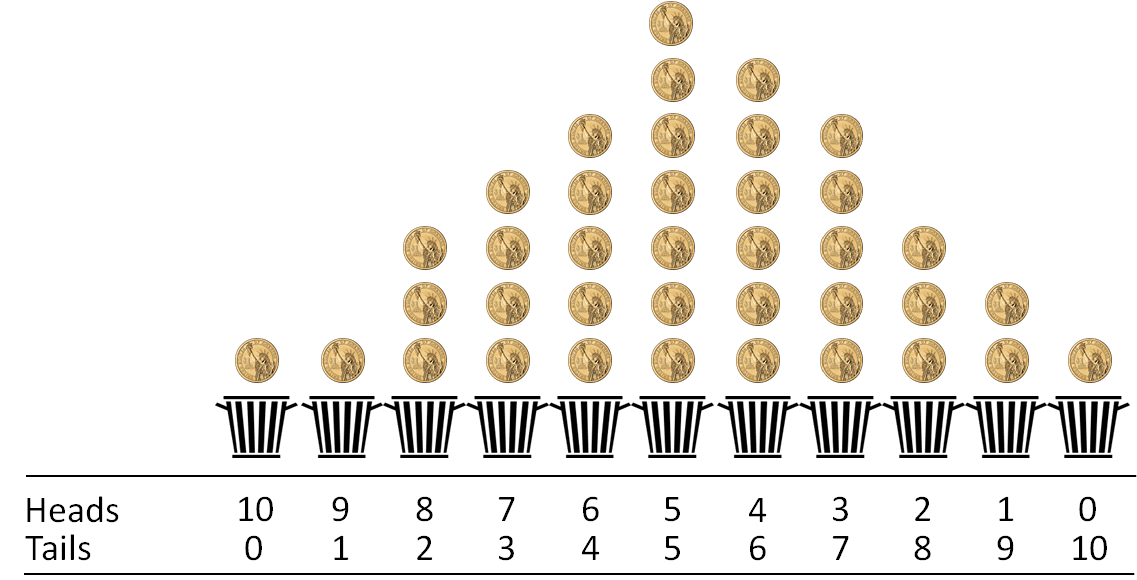​Now we're getting something that looks like a distribution!

We're not finished just yet, though, we've still got a couple of steps to go...

#### 4. ​Plotting a Histogram

​What we can do now is add up the tokens in each bin and plot them as a Histogram, which will give us something like this: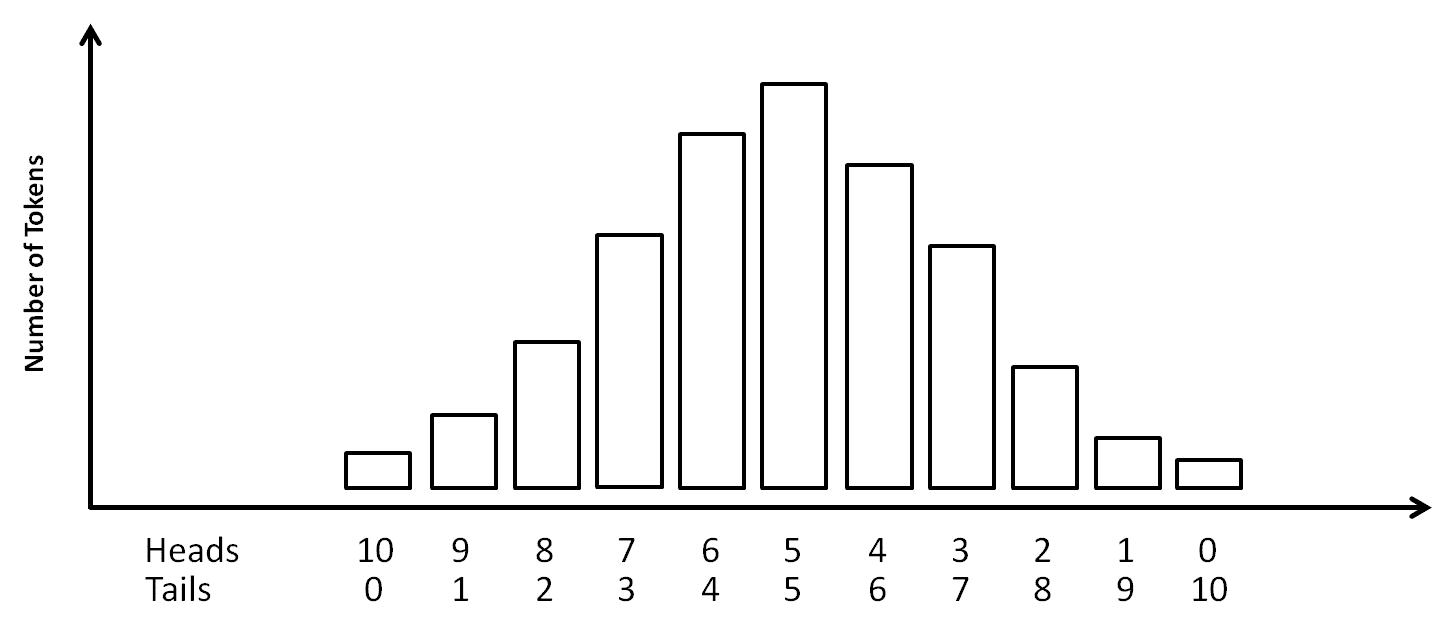​Now we're starting to look pretty good. We're not talking about coins, bins and tokens any more, we're now talking about axes and Histograms - much more professional!

But we still don't have a distribution yet. We're nearly there, but there's just one more step to go...

#### 5. ​Creating a Binomial Distribution

​If we now connect the tops of ​the bars of the Histogram and smooth them out with a magic smoothing algorithm, we'll get a nice smooth curve that sits on top of the Histogram.

This is the Binomial Distribution, and it looks like this: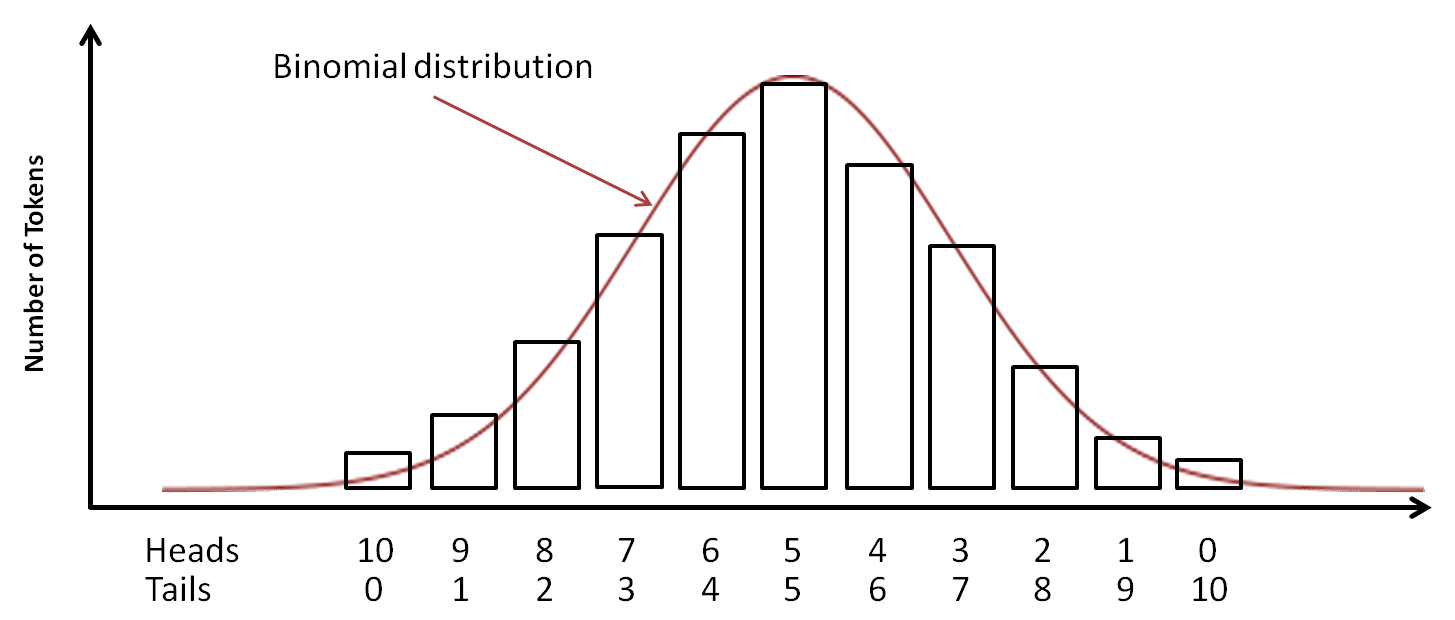​So now you know how to get a Binomial Distribution just by flipping a few coins.

And I hope you can see that the more coins you have, the more bins you have, and that the more times you repeat the experiment the smoother the ​Histogram becomes and looks more and more like the binomial curve.

OK, so now that we have a binomial distribution, what do we do with it?

Well, the first thing we can do is calculate the central point of our data, and how much spread our data has. For this, we would typically calculate the mean and standard deviation: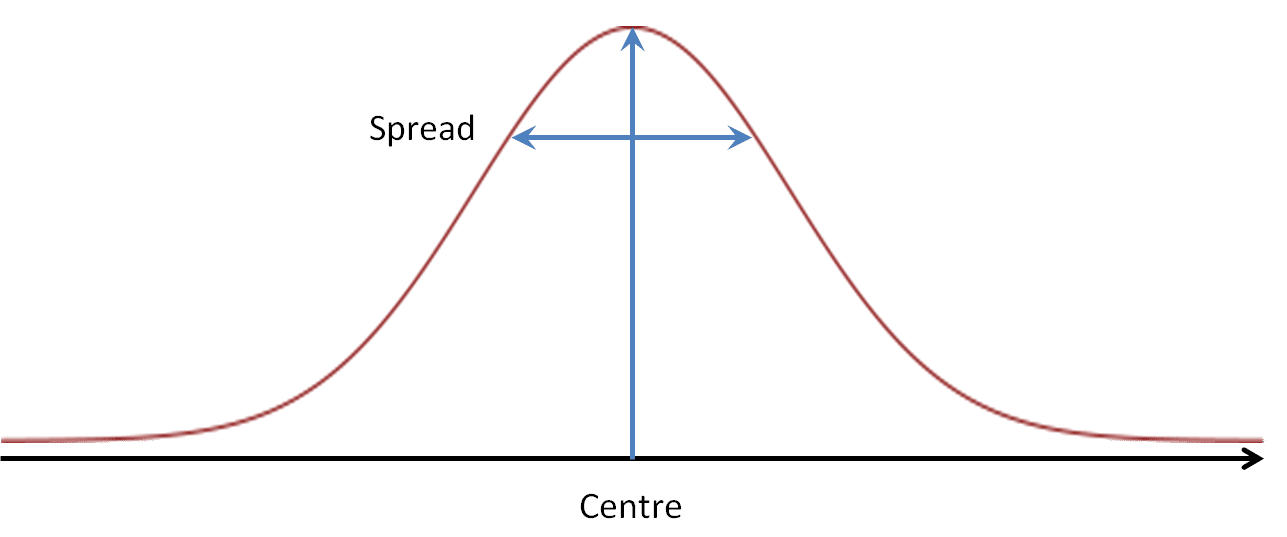​Once we have these 3 pieces of information [binomial distribution; mean; standard deviation], we have a way of ​describing all our data with just 2 numbers. That's pretty powerful!

Better still, since the binomial distribution is ​known​ (in other words, we have a mathematical equation that describes it), we have a way to compare what we expected to find (in theory, before we ran our experiment) with what we actually found (the results of the experiment).​​​

Our result might look like this: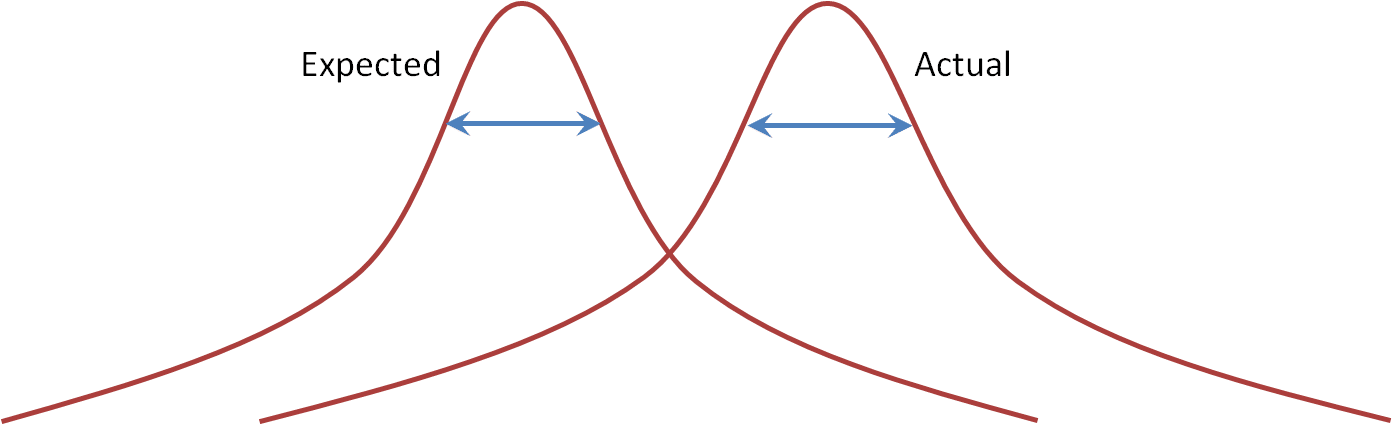​And from here we can do some fancy schmancy stats to see by how much our actual results differed from what we expected.

We can work out how extreme our results were, and from this express how likely our result occurred by chance, and this gives us an idea of whether our result is ​significant or not.

### ​The Binomial Distribution - A Summary

​The binomial distribution isn't some mythical creature sprinkled with magic pixie dust. It may be a bit of a mystery to some, but once you dive a little deeper you realise that it's not so tough after all.

Just by flipping a few coins and ​acknowledging that the order of the outcomes is unimportant, it becomes simple to realise that some outcomes are much rarer than others.

Plotting the outcomes gives us a way of identifying the common and the rarer results - the common results are always in the middle and the rarer results are always in the extremes.

Running lots of experiments wth lots of coins gives us a nice, neat graph that we call the Binomial Distribution.

And the binomial distribution is ​useful​.

We can use it to compare what we expect to find with what we actually find, and use it to tell us how likely it is that our result is important to us.

​​​The binomial distribution might not be sexy, but I reckon it's as cool as the Jamaican bobsled team...

I appreciate that ​the Binomial Distribution isn't the sexiest beast on the planet, so if you've got this far without running away, well done!

Give yourself a boost by clicking our 'Awesome Button'!

### Statistics - The Big Picture

I hope you enjoyed this video lesson.

It's actually one of the lessons in The Hive from our exclusive video course Statistics - The Big Picture, where I take you through all the nooks and crannies of the statistics world.

This course is Open Access to start with (you don't need to register), and after you've gone so far through you'll need a Free Plan to continue.

As part of the course you'll get an Ultra-HD pdf of Statistics - The Big Picture to download and keep, and I'll share with you where you can also get your own poster to pin on your wall.

If you want to continue learning, you can start from the beginning here:

### Statistics - The Big Picture

I look forward to seeing you on the inside!